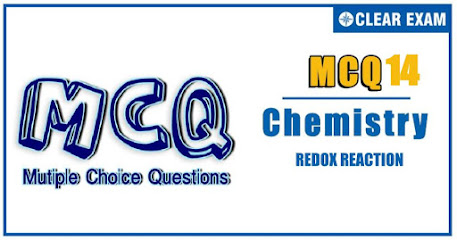## REDOX REACTION QUIZ-14

IIT JEE exam which consists of JEE Main and JEE Advanced is one of the most important entrance exams for engineering aspirants. The exam is held for candidates who are aspiring to pursue a career in the field of engineering and technical studies. Chemistry is important because everything you do is chemistry! Even your body is made of chemicals. Chemical reactions occur when you breathe, eat, or just sit there reading. All matter is made of chemicals, so the importance of chemistry is that it's the study of everything..

Solution
(c). LAH reduces ArNO_2 to (Ar-N=N-Ar) but does not reduce ArX (converts only 1°and 2° RX to RH and 3°RX to alkene). So the answer is (c)
Solution
(c). Proceed reverse: With O3/H2O, oxidative ozonolysis takes place

Solution
(c). (A)⇒HI + P reduces epoxides to alkanes (B) ⇒ LAH reduces expoxides to two different alcohols by cleaving the group from either side.

Q4. The oxidation number of in Pr in Pr6⁡O11 is
•  22/6
•  20/6
•  3
•  4
Solution
(a). Pr6O11: 6x-22=0 ∴x=22/6

Solution
(b). (A) must contain (MeCO-) group to give iodoform test. The reaction (C) to CH_3 COOH shows that (C) must be β-keto acid because on heating it easily undergoes decarboxylation, (or) (C) must be a dibasic acid. On heating it also loses CO_2. Therefore, (C) must be a dibasic acid because after iodoformreaction, (A) is converted to sodium salt of acid. So the answer is (b).

Solution
(a.) The isolated double bonds are not reduced by Birch reduction. So the answer is (a)

Solution
(d). Red P + HI reduces both alcohols and acids to alkanes. So the answer is (d).

Solution
(b). The reduction of ArNO_2 group under neutral conditions with (Zn dust +NH_4 Cl) or (Al-Hg + H_2 O)gives hydroxylamine compound. So the answer is (b).

Q9.In the reaction K+O2⟶KO2
•  O2 acts as an oxidizing agent
•  Both K and O2 are oxidized
•  O2 is oxidized while K is reduced
•  K acts as an oxidizing agent
Solution
(a). K⟶K^⊕+e^- (oxidation,acts as reducing agent) e^-+O_2⟶O_2^(1-) (reduction,acts as oxidizing agent)

Q10. Which of the following is not a redox reaction?
•  CaCO3⟶CaO+CO2
•  O2+2H2⟶2H2O
• Na+H2O⟶NaOH+1/2H2
• MnCl3⟶MnCl2+(1/2)Cl2
Solution
(a). a. Oxidation states of Ca and C are +2 and +4, respectively, in both reactant and product: hence, not redox, b.4e^-+O_2⟶2O^(2-) (Reduction) H_2⟶2H^⊕+2e^- (Oxidation) Hence, redox c. Na ⟶Na^⊕+e^- (Oxidation) 2H^⊕+2e^-⟶H2 (Reduction) Hence, redox d. e^-+Mn^(3+)⟶Mn^(2+) (Reduction) 2Cl^⊖⟶Cl2+2e^-(Oxidation) Hence, redox#### Written by: AUTHORNAME

AUTHORDESCRIPTION## Want to know more

Please fill in the details below:

## Latest NEET Articles\$type=three\$c=3\$author=hide\$comment=hide\$rm=hide\$date=hide\$snippet=hide

Name

ltr
item
BEST NEET COACHING CENTER | BEST IIT JEE COACHING INSTITUTE | BEST NEET & IIT JEE COACHING: REDOX REACTIONS Quiz-14
REDOX REACTIONS Quiz-14